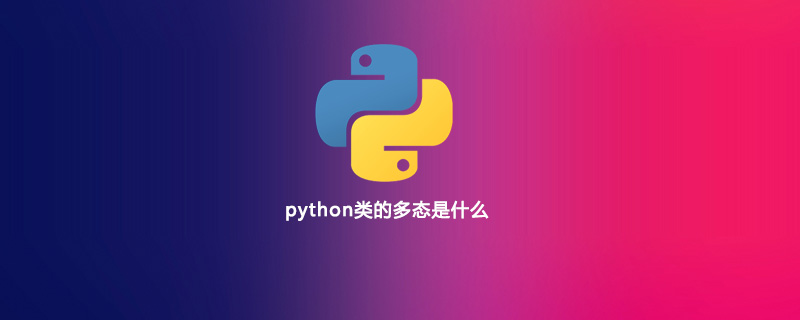# python类的多态是什么```Fu f1 = new Zi1();
Fu f2 = new Zi2();
f1.c();
f2.c();```

```#!/usr/bin/env python3
# -*- coding:utf-8 -*-
class Person:
def __init__(self, name, age):
self.name = name
self.age = age
def print_age(self):
print("%s's age is %s" % (self.name, self.age))
class Man(Person):
def print_age(self):
print("Mr. %s's age is %s" %(self.name, self.age))
class Woman(Person):
def print_age(self):
print("Ms. %s's age is %s" %(self.name, self.age))

def person_age(person):
person.print_age()
person = Person("kevin", 23)
man = Man("Bob", 33)
woman = Woman("Lily", 28)
person_age(person)
person_age(man)
person_age(woman)```

```kevin's age is 23
Mr. Bob's age is 33
Ms. Lily's age is 28```

Python开发开发项目